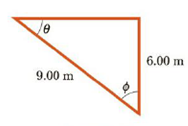Chapter 1, Problem 45P

Chapter
Section
Textbook Problem

For the triangle shown in Figure P1.45, what are (a) the length of the unknown side, (b) the tangent of θ, and (c) the sine of Φ?Figure P1.45

(a)

To determine
The length of the unknown side of the right triangle.

Explanation

Given Info: The length of the other two side of the right triangle is a=6m and hypotenuse c=9m

Explanation:

The diagram for the right triangle is,

Formula to calculate the length of the other side of the right triangle is,

b=c2a2

• b is other side of the right triangle,
• c is the hypotenuse of the right triangle,
• a is the another side of the right triangle

Substitute 9m for c , 6m for a to find b

(b)

To determine
The tangent of θ .

(c)

To determine
The sine of ϕ .

Still sussing out bartleby?

Check out a sample textbook solution.

See a sample solution

The Solution to Your Study Problems

Bartleby provides explanations to thousands of textbook problems written by our experts, many with advanced degrees!

Get Started